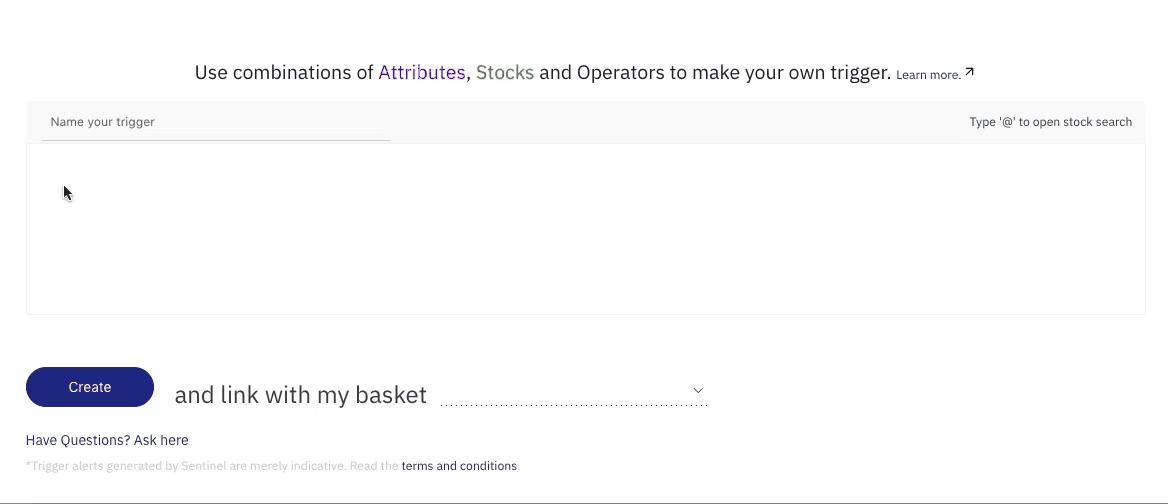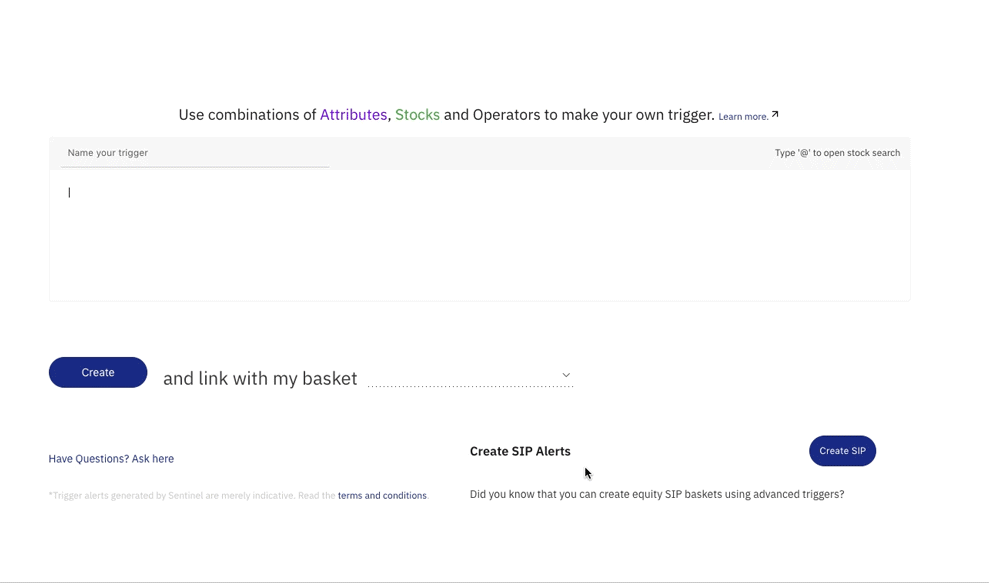Sentinel Pro lets you write Advanced Triggers in a simple language resembling Excel formulas and arithmetic expressions. This allows the creation of powerful triggers combining multiple stocks and instruments and mathematical conditions.For instance, it's possible to create a trigger that alerts when the close price of `INFY` is 2% less than the opening price of an `INFYFUT` and the NIFTY 50 index, or any such combination. This tutorial runs you through the Sentinel Trigger Language.

Sentinel Triggers evaluate to a boolean value always. Whenever you create a new trigger, it should evaluate to `False` at that instant, only then the trigger is queued in our trigger evaluation engine. When a queued trigger is evaluated to `True` an alert is generated to the end user and the trigger is popped from the queue.

For instance, assuming `INFY` is currently trading at 700, the condition:

```LastTradedPrice('NSE:INFY') >= 800
```

will evaluate to `False` as of now. When `INFY` touches or crosses 800, whenever that is in the future, the trigger will evaluate to `True`, thereby generating an alert.

## Functions¶

Function refers to the properties of an instrument. The functions are divided into the following 3 categories

• Instrument Functions
• Date Functions
• Math Functions

### Instrument Functions¶

Function Description
HighPrice('exchange:tradingsymbol') High price for the day
LowPrice('exchange:tradingsymbol') Low price for the day
DayChange('exchange:tradingsymbol') Absolute change in price compared to previous day's market close
DayChangePercent('exchange:tradingsymbol') Percentage change in price compared to previous day's market close
TotalSellQuantity('exchange:tradingsymbol') Total number of sell orders for the day
OpenInterest('exchange:tradingsymbol') Open Interest (OI) for the F&O contracts

### Date - Time Functions¶

Function Description
Year() Current year component of timestamp (eg: 2019, 2020)
Month() Current month of the year (1-12)
Day() Current day of the month (1-31)
Hour() Current hour of the day (0-23)
Minute() Current minute of the day (0-59)
Second() Current second of the day (0-59)
YearDay() Current day of the year (1-365)
Weekday() Current day of the week (0-6)
Week() Current week of the year (1-53)
Timestamp() Current time (IST) representation in ISO 8601 format
DateString() String representation of time in 'yyyy-mm-dd hh:mm:ss' or 'yyyy-mm-dd' format

### Math Functions¶

Function Description
Math_Abs(number) Absolute value of a number
Math_Ceil(number) Least integer value greater than or equal to a number
Math_Floor(number) Greatest integer value less than or equal to a number
Math_Avg(number1, number2, ...) Average value of all numbers
Math_Max(number1, number2, ...) Largest value of all numbers
Math_Min(number1, number2, ...) Smallest value of all numbers
Math_StdDev(number1, number2, ...) Standard deviation value of all numbers
Math_Exp(number) Base-e exponential of number, i.e. e**x
Math_Exp2(number) Base-2 exponential of number, i.e. 2**x
Math_Log(number) Natural logarithm of a number
Math_Log2(number) Decimal logarithm of a number
Math_Log10(number) Decimal logarithm of a number with base 10
Math_Round(number) Round returns the nearest integer to number, rounding half away from zero

## Operators¶

Operator refers to the mathematical constructs which are used to compare or bind two values, such as "equal to", "greater than" etc. These are the building blocks for conditions in triggers.

Operator Description
`==` Equal to
`!=` Not equal to
`<` Lesser than
`<=` Lesser than or equal to
`>` Greater than
`>=` Greater than or equal to
`&&` Logical AND operator
`||` Logical OR operator

## Understanding Syntax¶

The format is simple - you can set conditions for alerts using functions and comparing them using operators

The Syntax for a function is: `Function('EXCHANGE:TRADINGSYMBOL')`. For example:

`LastTradedPrice('NSE:INFY')`

`OpenInterest('NFO:NIFTY18MAYFUT')`

To form more complex triggers, these function blocks can be compared using arithmetic operators, the format is:

```LastTradedPrice('NSE:INFY') > LastTradedPrice('NFO:INFY18MAYFUT')
```

To set an Advanced trigger, go to dashboard and click on Advanced triggers. In this page, you will see an editor field where you can write your triggers.

In the editor, write the function based on which you want the alert. You don’t have to type the whole function, as you start typing the first few alphabets, Sentinel will autosuggest the function. You can select any of the function by hitting the Enter key.

After selecting the function, type the trading symbol for the instrument, auto-suggestion will be applicable here as well, hit the Enter key to select the scrip. You can choose the operator and continue writing the remaining part of the trigger in the same way.

Once you write your trigger, give it a name in the Name your trigger field and click on Create. You will be able to see all your active triggers under My triggers on the dashboard.

You can now invest systematically (SIP) in stocks and ETFs on Sentinel with the new SIP alerts feature. You can create weekly, fortnightly, or monthly alerts as a reminder for you to invest and tag a basket of stocks. When you get the alert, you can execute the basket order in just one click.## Example Triggers¶

The most fundamental unit of a trigger is of the form `Function('EXCHANGE:TRADINGSYMBOL')`. For example:

```LastTradedPrice('NSE:INFY') or OpenInterest('NFO:NIFTY18MAYFUT')
```

To form more complex rules, these units can be compared using arithmetic operators, for example:

```LastTradedPrice('NSE:INFY') > LastTradedPrice('NFO:INFY18MAYFUT)
```

They can be mixed with constant numbers and arithmetic operators. To group logical blocks, they are wrapped with opening parenthesis `(` and closing parenthesis `)`.

```LastTradedPrice('NSE:INFY') / 2) > (LastTradedPrice('NFO:INFY18MAYFUT') * 0.5)
```

Such blocks can be further combined with logical operators to evaluate multiple conditions. The following trigger evaluates to true if the LastTradedPrice of INFY has dropped below its day's ClosePrice `OR` if the same condition occurs for RELIANCE

```LastTradedPrice('NSE:INFY') < ClosePrice('NSE:INFY') || (LastTradedPrice('NSE:RELIANCE') < ClosePrice('NSE:RELIANCE')
```

The triggers can also be combined with logical operators to evaluate only if it meets the date conditions as well. The following trigger evaluates to true if the LastTradedPrice of INFY has crossed above 1500 and the current date is after 5th January 2019

```LastTradedPrice('NSE:INFY') > 1500 && Timestamp() > DateString('2019-01-05')
```

The triggers can be set to trigger only on certain time intervals with date conditions. The following trigger evaluates to true if the LastTradedPrice of INFY has crossed above 1500 and the day of the month is 3rd or beyond 3rd. This would mean that even if INFY crossed 1500 on 1st of the month, the trigger will not evaluate to false since the date condition of the day being 3rd or beyond 3rd also needs to be satisfied.

```LastTradedPrice('NSE:INFY') > 1500 && Day() >= 3
```

Sentinel Trigger Language also has utility for math functions which can be useful while creating triggers. The following trigger evaluates to true if the Average of Open of INFY and Close of INFY has crossed above 1200

```Math_Avg(OpenPrice('NSE:INFY'), ClosePrice('NSE:INFY')) > 1200
```

These math functions can be of great use if you want to create triggers based on a mathematical formula. For example, if you want to calculate Typical Price of an instrument, the formula used is Average of HighPrice, LowPrice, ClosePrice. For example, you want to create a trigger which alerts when Typical Price of INFY crosses above 900, you can simply write

```Math_Avg(HighPrice('NSE:INFY'), ClosePrice('NSE:INFY'), LowPrice('NSE:INFY')) > 900
```